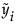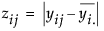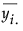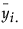where n represents the number of yijk observations.
Brown-Forsythe is the model F statistic from an ANOVA onwhereis the median response for the ith level.
The Levene F is the model F statistic from an ANOVA onwhereis the mean response for the ith level.
and ni is the count on the ith level and si2 is the response sample variance on the ith level. The Bartlett statistic has a χ2-distribution. Dividing the Chi-square test statistic by the degrees of freedom results in the reported F value.
and ni is the count on the ith level,is the mean response for the ith level, and si2 is the response sample variance for the ith level.
where wi, ni, and u are defined as in the F ratio formula.

Help created on 9/19/2017